﻿ Mechanical Oscillation of the Cam Mechanism

Mechanical Oscillation of the Cam Mechanism

Peter Frankovský, Ingrid Delyová, Peter Sivák, Darina Hroncová, Marek Výrostek

American Journal of Mechanical Engineering

Mechanical Oscillation of the Cam Mechanism

Peter Frankovský1,, Ingrid Delyová2, Peter Sivák2, Darina Hroncová1, Marek Výrostek3

1Department of Mechatronics, Faculty of Mechanical Engineering Technical University of Košice, Slovakia

2Department of Applied Mechanics and Mechanical Engineering, Faculty of Mechanical Engineering Technical University of Košice, Slovakia

3Zastrova a.s., Spišská Stará Ves, Slovakia

Abstract

This article deals with the dynamic analysis of cam mechanism using MSC Adams. In the first part of this paper is solving the dynamic analysis using the Newtonian mechanics. The mathematical model was designed by using this method of dynamic analysis. The second part deals with the dynamic analysis in the program MSC Adams/View. The dynamic analysis is important in terms usability of cam mechanism.

• Peter Frankovský, Ingrid Delyová, Peter Sivák, Darina Hroncová, Marek Výrostek. Mechanical Oscillation of the Cam Mechanism. American Journal of Mechanical Engineering. Vol. 3, No. 6, 2015, pp 207-210. http://pubs.sciepub.com/ajme/3/6/10
• Frankovský, Peter, et al. "Mechanical Oscillation of the Cam Mechanism." American Journal of Mechanical Engineering 3.6 (2015): 207-210.
• Frankovský, P. , Delyová, I. , Sivák, P. , Hroncová, D. , & Výrostek, M. (2015). Mechanical Oscillation of the Cam Mechanism. American Journal of Mechanical Engineering, 3(6), 207-210.
• Frankovský, Peter, Ingrid Delyová, Peter Sivák, Darina Hroncová, and Marek Výrostek. "Mechanical Oscillation of the Cam Mechanism." American Journal of Mechanical Engineering 3, no. 6 (2015): 207-210.

 Import into BibTeX Import into EndNote Import into RefMan Import into RefWorks

12345
Prev Next

1. Introduction

The cam mechanisms have been used for a long time until today and are included as an integral part of combustion engines. They are encountered in installations where comes transformation of rotary movement to movement of shifting in practice. The advantage of cam mechanisms is their simplicity and high functionality. The dynamic analysis of the mechanism with a circular cam is the aim of this work. This analysis is necessary when dealing with design of a cam mechanism it to achieve the best result which means to achieve desired properties when is used the smallest amount of material, for instance.

Mathematical model of the cam mechanism was derived by using Newton’s dynamics. In the second part of the dynamic analysis is solution by program MSC Adams. Programs such as MSC Adams can facilitate dynamic analysis of various complex mechanisms which simplify and velocity up the analysis results. To the desired results solutions leads correctly preparation of dimensional model in a computer program simulation of MSC. That program allows you to evaluate the results in graphical and numerical form. It can determine kinematics quantities such as: displacement, velocity, acceleration, and other physical quantities. The dynamic analysis is important in terms usability of the cam mechanism, because of not every shape and the characteristics of that cam meets the specific mechanism. The graphical course of displacement, velocity and acceleration is suggested to show us the correct function of cam mechanism.

2. Cam Mechanism and Newtonian Mechanics

Our task is to deal with the motion of matter. We need to determine the force transmitted to the resilient member. On the figure is shown the equivalent circuit of cam mechanism with consideration to the follower part with stiffness k. We applied Newton's equations in examining the absolute motion of the mass m in form :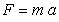(1)

To analyze the vibration, we shall consider the mass m of the cam mechanism shown in the position in Figure 1.Download asVeiw figureFigures index
Figure 1. The cam mechanism model

The force exerted by the spring in the position in Figure 1  has a magnitude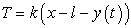. Substituting for F into the fundamental equation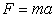and recalling that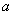is the second derivative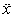of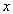with respect to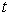, we write: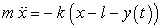(2)

or we write in the form: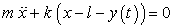(3)

and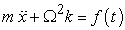(4)

where:

Ω the size of angular velocity, circular frequency of the vibration.

Function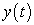is given by the stroke dependence of the cam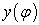and rotational movement of the cam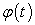. In the uniform rotation of the camshaft is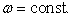,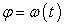and the course of movementswill be periodic functions of time. If we explicate this periodic function with period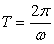to the Fourier series, we get: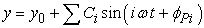(5)

Equation of the motion we write in the form: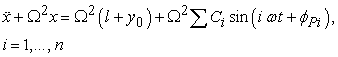(6)

The stationary part of the oscillation is then given by equation in form: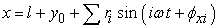(7)

where:

To calculate the force transmitted by the spring we determine the course of deformation ξ (t). It is the examination of the relative motion, the equation of motion is: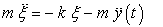(8)

after that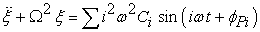(9)

The stationary part of the solution is then given in form: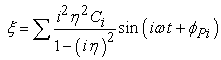(10)

The force of the spring is then given by equation in form: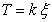(11)

This is not only one method of analysis of dynamics which is so difficult; there are also others where solution of movement equation of cam mechanism is not so easy and demanding. Therefore, the use of the program MSC Adams is very advantageous .

3. Using MSC Adams/View

With MSC Adams software users can produce virtual prototypes to simulate complex mechanical systems and quickly analyze multiple design variations until an optimal design is achieved. MSC Adams is one of many computer programs for modeling and simulating multi body systems. In programs a virtual prototype of a mechanism show in Figure 2 and Figure 3 can be built and then simulated .

If the results, a certain movement or load transmission are not satisfy, the prototype can easily be modified and then simulated again.

Adams is the world’s most widely used multi-body dynamics simulation software. It lets you build and test virtual prototypes, realistically simulating on your computer, both visually and mathematically, the full–motion behavior of your complex mechanical system designs as shown in the Figure 3.Download asVeiw figureFigures index
Figure 2. The cam mechanism model in MSC Adams/ViewDownload asVeiw figureFigures index
Figure 3. The cam mechanism – spatial view

The software checks your model and automatically formulates and solves the equations of motion for kinematical or dynamic simulations.Download asVeiw figureFigures index
Figure 4. The translational displacement, velocity and acceleration of the PART_2 camDownload asVeiw figureFigures index
Figure 5. The translational displacement and velocity of the PART_3Download asVeiw figureFigures index
Figure 6. The translational displacement and velocity of the PART_4

After construction model in MSC Adams/View shown in Figure 2 we could try to simulate the movement of the cam mechanism and to determine the physical properties .

The Post Processor processes the results of the solution position, velocity, acceleration of the part_2 (Figure 4), position, velocity of the part_3 (Figure 5), position and velocity of the part_4 (Figure 6).

We have chosen necessary interested variables such as displacement, velocity and acceleration. The next step was to choose the correct folder where variables are investigated. Graphs of the spring deformation and spring force using the MSC Adams program are shown in next figures .Download asVeiw figureFigures index
Figure 7. The Windows of the measures in MSC Adams/View

The Postprocessor provides complete value at certain time intervals and of course the specific waveforms from which can be read values .Download asVeiw figureFigures index
Figure 8. The postprocessor window with the animation model and parameters of the spring

The last step of dynamic analysis of cam mechanism was to find out some forces which operate in the spring when is the cam mechanisms in motion. The size and the course of force of spring are shown in Figure 8 and Figure 9 [6, 7].Download asVeiw figureFigures index
Figure 9. The postprocessor window with the animation model and record in format avi

The necessary interested variables such as displacement and velocity of the cam are shown in Figure 10 and Figure 11.Download asVeiw figureFigures index
Figure 10. The translational displacement of the PART_2 camDownload asVeiw figureFigures index
Figure 11. The translational velocity of the PART_2 cam

The course of deformation of the spring with respect to time is shown in Figure 12.Download asVeiw figureFigures index
Figure 12. Spring deformation of the mechanismDownload asVeiw figureFigures index
Figure 13. Spring force of the mechanism

The course of the spring force with respect to time is shown in Figure 13.

4. Conclusion

In this paper one module of the number of modules namely MSC Adams/View was used as a tool which allows a better simulation and visualization of the model and easier evaluation of the results obtained. With the module MSC Adams/View they represent a tool that is able to addresses various types of mechanisms with many degrees of freedom.

In this article it is shown a procedure for solving kinematics problem of the mechanism using modeling in program MSC Adams View. This program allows simulate moving of such mechanical systems. Results are obtained in form of time diagram of the desired variables. Tasks are solved numerically. Model is compiled by using program MSC Adams View . The results are obtained by the simulation of cam mechanism were processed by the postprocessor program of MSC Adams/View. Program MSC Adams/View makes it easy to analyze complex mechanical systems with multiple degrees of freedom.

Mastering this methodology provides a suitable tool for solving problems of teaching and practice. The contribution of this work is mainly didactic. Especially in the field of Applied Mechanics, presents the possibility of computer simulation.

Acknowledgement

This work was supported by the Ministry of Education of the Slovakia Foundation under Grant VEGA No. 1/1205/12 and VEGA No. 1/0937/12.

References

  Bocko, Jozef; Frankovský, Peter; Delyová, Ingrid; Pástor, Miroslav; Kinematika v príkladoch, SjF TU v Košiciach, 2011.In article  Brát, Vladimír, Brousil, Otakar: Dynamika. Praha: ČVUT, 1989.In article  Frankovský, Peter; Delyová, Ingrid; Hroncová, Darina; “Kinematic analysis of movement of a point of a simple mechanism”, 4th International Conference Modelling of mechanical and mechatronics systems, KAMaM, Technical university Košice, Herľany, 2011, Slovakia, s.53-58.In article PubMed  Hroncová, Darina; Binda, Michal; Šarga, Patrik; Kičák, František; “Kinematical Analysis of Crank Slider Mechanism Using MSC AdamsView”, In: Procedia Engineering: MMaMS 2012: Modelling of Mechanical and Mechatronics Systems 2012: November 6th-8th 2012, Zemplínska Šírava, Slovakia. Košice: TU, 2012 Vol. 48, p. 213-222.In article  https://tech.purdue.edu/plm/SME/product_design.phpIn article  http://www.mscsoftware.com/product/adamsIn article  Kollár, P., Nikitin, Y., Straka, M.: The determination of the shelf mass in the universal shelving stacker by measuring the frequency converter torque generating current of the main drive, Manufacturing technology, Vol. 15, No. 3, p. 363-366, ISSN 1213-2489.In article